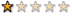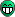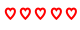## [問題] The Blog Mod時間調整

phpBB 2 MOD Support

(發表文章請按照公告格式發表，違者砍文)### [問題] The Blog Mod時間調整

http://www.phpbb.com/phpBB/viewtopic.ph ... t=album+cp

http://lyn.in-tw.com

Arisa520http://d-s.idv.tw:88/phpbb/weblogs.phpTimezone problem:

(removed)
↑似乎是把時區問題的語法給移除了..........QQ
yll``````if ( isset(\$HTTP_POST_VARS['submit']) )
{
\$target_date = mktime(\$hour, \$minute, \$second, \$month, \$day, \$year);

\$target_date += (3600 * \$board_config['board_timezone']);

\$hour = date("G", time());
\$minute = date("i", time());
\$second = date("s", time());
\$year = date("Y", \$target_date);
\$month = date("n", \$target_date);
\$day = date("j", \$target_date);
}``````

``\$target_date += (3600 * \$board_config['board_timezone']);``

``// \$target_date += (3600 * \$board_config['board_timezone']);``
okDemo:
http://yll.loxa.edu.tw/phpBB2/weblogs.php
timkuanArisa520 寫:他的官方網站上已經有修正~

http://d-s.idv.tw:88/phpbb/weblogs.php

timkuan@gmail.comyll 寫:打開weblog_posting.php

``````if ( isset(\$HTTP_POST_VARS['submit']) )
{
\$target_date = mktime(\$hour, \$minute, \$second, \$month, \$day, \$year);

\$target_date += (3600 * \$board_config['board_timezone']);

\$hour = date("G", time());
\$minute = date("i", time());
\$second = date("s", time());
\$year = date("Y", \$target_date);
\$month = date("n", \$target_date);
\$day = date("j", \$target_date);
}``````

``\$target_date += (3600 * \$board_config['board_timezone']);``

``// \$target_date += (3600 * \$board_config['board_timezone']);``
okDemo:
http://yll.loxa.edu.tw/phpBB2/weblogs.php

``````#
#-----[ Open ]-----
#
includes/functions_weblog.php

#
#-----[ Find ]-----
#
AND e.entry_time <= " . time() . "

#
#-----[ Replace With ]-----
#
AND e.entry_time <= " . (time() + (3600 * \$board_config['board_timezone']) ) . "

#
#-----[ Save & Close ]-----
#
#EoM
``````
Source: http://vince.dynalias.com/blog/viewtopic.php?p=716#716

ps. 原作者回答時, 恐有筆誤.

``````AND e.entry_time < " . time() . "
``````

http://wang5555.hopto.org/phpBB3/
p.s. 奉老婆之命：在晚上十一點前，得關機睡覺！！！yll 寫:打開weblog_posting.php

``````if ( isset(\$HTTP_POST_VARS['submit']) )
{
\$target_date = mktime(\$hour, \$minute, \$second, \$month, \$day, \$year);

\$target_date += (3600 * \$board_config['board_timezone']);

\$hour = date("G", time());
\$minute = date("i", time());
\$second = date("s", time());
\$year = date("Y", \$target_date);
\$month = date("n", \$target_date);
\$day = date("j", \$target_date);
}``````

``\$target_date += (3600 * \$board_config['board_timezone']);``

``// \$target_date += (3600 * \$board_config['board_timezone']);``
okDemo:
http://yll.loxa.edu.tw/phpBB2/weblogs.php

``````#
#-----[ Open ]-----
#
includes/functions_weblog.php

#
#-----[ Find ]-----
#
AND e.entry_time <= " . time() . "

#
#-----[ Replace With ]-----
#
AND e.entry_time <= " . (time() + (3600 * \$board_config['board_timezone']) ) . "

#
#-----[ Save & Close ]-----
#
#EoM
``````
Source: http://vince.dynalias.com/blog/viewtopic.php?p=716#716

ps. 原作者回答時, 恐有筆誤.

``````AND e.entry_time < " . time() . "
``````To 好手:

(ps. 將它另存成文字檔後, 再貼出下載路徑即可)

http://wang5555.hopto.org/phpBB3/
p.s. 奉老婆之命：在晚上十一點前，得關機睡覺！！！